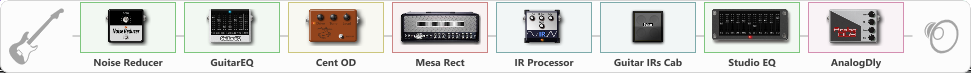# RECTIFIED

Discussion in 'ToneLib-GFX presets' started by LINDSAY ROSS, Oct 1, 2021.

1. RECTIFIED

Preset name: BOOGIE RECTIFIER

Effects chain:Effect: "Noise Reducer" (Dynamics / Filter), active - "yes"
{
"Sens" = 100
"Mode" = Hard
}

Effect: "GuitarEQ" (Dynamics / Filter), active - "yes"
{
"160 Hz" = 3
"400 Hz" = -6
"800 Hz" = 0
"1.6 kHz" = -4
"3.2 kHz" = -7
"6.4 kHz" = -7
"12 kHz" = -2
"Level (dB)" = 2
}

Effect: "Cent OD" (Overdrive / Distortion), active - "yes"
{
"Drive" = 38
"Tone" = 44
"Level" = 38
}

Effect: "Mesa Rect" (Amp simulators), active - "yes"
{
"Gain" = 28
"Bass" = 42
"Middle" = 43
"Treble" = 50
"Presence" = 16
"Master" = 50
"Level (dB)" = 6
}

Effect: "IR Processor" (Cabinets), active - "yes"
{
"IR" = PRS DRIVE
"Low Cut (Hz)" = 0
"Hi Cut (kHz)" = 20.0
"Mix" = 100
"Level (dB)" = 0
}

Effect: "Guitar IRs Cab" (Cabinets), active - "yes"
{
"Model" = Fender Twin Rev (2x12")
"Mic Position" = Middle
"Mic Distance" = Middle
"Low Cut (Hz)" = 95
"Hi Cut (kHz)" = 20.0
"Mix" = 100
"Level (dB)" = 0
}

Effect: "Studio EQ" (Dynamics / Filter), active - "yes"
{
"31 Hz" = 0
"62 Hz" = 0
"125 Hz" = 3
"250 Hz" = 2
"500 Hz" = -1
"1 kHz" = -4
"2 kHz" = -5
"4 kHz" = -7
"8 kHz" = -11
"16 kHz" = -13
"Level (dB)" = 0
}

Effect: "AnalogDly" (Delay), active - "yes"
{
"Time" = 680
"Feedback" = 18
"Tone" = 25
"Mix" = 53
}

Note: You will need to download and install the ToneLib-GFX software to use the preset.

#### Attached Files:

• ###### RECTIFIED.tlgfx
File size:
3.8 KB
Views:
2,213
ktaxi likes this.